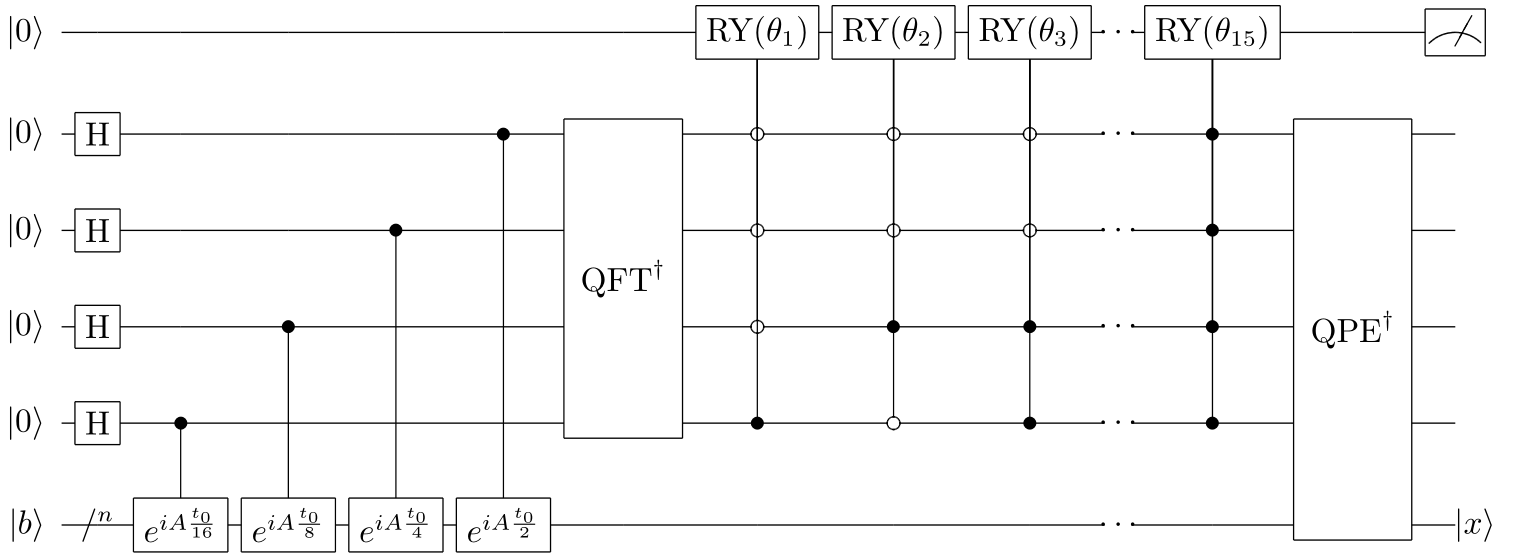# HHL算法¶

HHL算法是一种求解线性方程组的量子算法，线性方程组在许多领域中都有着广泛的实际应用。

## 问题背景概述¶

HHL算法相对于经典算法有着指数级的加速，但经典算法可以返回精确解，而HHL算法只能返回近似解。

HHL算法是一种纯量子算法，它和它的改进版的出现对于证明量子算法的实用性有着重大意义。

## 算法原理¶

1. 构造右端项量子态，对存储比特及右端项比特进行参数含左端项矩阵的相位估计，将左端项矩阵的整数形式特征值全部转移到存储比特的基向量中。
2. 进行一系列参数含特征值的受控旋转，过滤出所有的特征值相关量子态，将特征值从存储比特的基向量转移到振幅；
3. 对特征存储比特及右端项比特进行逆相位估计，将存储比特振幅上的特征值合并到右端项比特上，当辅助比特测量得到特定状态时，在右端项比特上可得到解的量子态。

\begin{split}\begin{aligned} C_A=\left[\begin{matrix}0&A\\A^H&0\\\end{matrix}\right], C_b=\left[\begin{matrix}b\\0\\\end{matrix}\right], C_x=\left[\begin{matrix}0\\x\\\end{matrix}\right], \end{aligned}\end{split}

\begin{aligned} A=\sum_{j=0}^{N-1}\lambda_j\left|u_j\right\rangle\left\langle u_j\right|,\lambda_j\in R. \end{aligned}

$$\left|b\right\rangle$$ 以特征向量基展开，得到

\begin{aligned} \left|b\right\rangle=\sum_{j=0}^{N-1}{b_j\left|u_j\right\rangle},b_j\in C. \end{aligned}

$\left|x\right\rangle=A^{-1}\left|b\right\rangle=\sum_{j=0}^{N-1}{\lambda_j^{-1}b_j\left|u_j\right\rangle.}$

### 通过QPE提取特征值¶

$$\left|0\right\rangle^{\otimes n}\left|b\right\rangle$$ 进行一次QPE操作，得到

\begin{aligned} {QPE(\left|0\right\rangle}^{\otimes n}\left|b\right\rangle)=\sum_{j=0}^{N-1}{b_j\left|\widetilde{\lambda_j}\right\rangle\left|u_j\right\rangle}. \end{aligned}

### 通过受控旋转转移特征值¶

\begin{split}\begin{aligned} CR(k)(\left|a\right\rangle\left|j\right\rangle)=\left\{\begin{matrix} RY(\arccos{\frac{C}{k}})\left|a\right\rangle\left|k\right\rangle,j=k,\\ \left|a\right\rangle\left|j\right\rangle,j\neq k, \end{matrix}\right. \end{aligned}\end{split}

\begin{aligned} (\prod (CR(k)\otimes I))\sum_{N-1}^{j=0}b_j\left|0\right\rangle\left|\widetilde{\lambda_j}\right\rangle \left|u_j\right\rangle=\sum_{j=0}^{N-1}{(\sqrt{1-\frac{C^2}{{\widetilde{\lambda_j}}^2}}\left|0\right\rangle +\frac{C}{\widetilde{\lambda_j}}\left|1\right\rangle)b_j\left|\widetilde{\lambda_j}\right\rangle\left|u_j\right\rangle}. \end{aligned}

### 通过逆QPE输出结果量子态¶

\begin{split}\begin{aligned} & (I\otimes{QPE}^{\dagger})\sum_{j=0}^{N-1}{(\sqrt{1-\frac{C^2}{{\widetilde{\lambda_j}}^2}}\left|0\right\rangle+\frac{C}{\widetilde{\lambda_j}} \left|1\right\rangle)b_j\left|\widetilde{\lambda_j}\right\rangle\left|u_j\right\rangle} \\ & =\sum_{j=0}^{N-1}{(b_j}\sqrt{1-\frac{C^2}{{\widetilde{\lambda_j}}^2}}\left|0\right\rangle\left|0\right\rangle\left|u_j\right\rangle+b_j \frac{C}{\widetilde{\lambda_j}}\left|1\right\rangle\left|0\right\rangle\left|u_j\right\rangle). \end{aligned}\end{split}

HHL算法充分利用了量子相位估计提取特征值信息的功能，巧妙构造了受控旋转门从存储比特的基向量中抓取特征值存入振幅， 最后利用逆相位估计还原存储量子比特，从而得到了振幅含特征值的方程解。

## 量子线路图与参考代码¶

HHL算法的量子线路图如下所示QCircuit build_HHL_circuit(const QStat& A, const std::vector<double>& b, QuantumMachine *qvm);
QStat HHL_solve_linear_equations(const QStat& A, const std::vector<double>& b);


#include "QPanda.h"
using namespace QPanda;

int main(void)
{
auto machine = initQuantumMachine(CPU);
auto prog = QProg();

QStat A = {
qcomplex_t(15.0 / 4.0, 0), qcomplex_t(9.0 / 4.0, 0), qcomplex_t(5.0 / 4.0, 0), qcomplex_t(-3.0 / 4.0, 0),
qcomplex_t(9.0 / 4.0, 0), qcomplex_t(15.0 / 4.0, 0), qcomplex_t(3.0 / 4.0, 0), qcomplex_t(-5.0 / 4.0, 0),
qcomplex_t(5.0 / 4.0, 0), qcomplex_t(3.0 / 4.0, 0), qcomplex_t(15.0 / 4.0, 0), qcomplex_t(-9.0 / 4.0, 0),
qcomplex_t(-3.0 / 4.0, 0), qcomplex_t(-5.0 / 4.0, 0), qcomplex_t(-9.0 / 4.0, 0), qcomplex_t(15.0 / 4.0, 0)
};

std::vector<double> b = { 0.5, 0.5, 0.5, 0.5 };

QStat result = HHL_solve_linear_equations(A, b);
int w = 0;
double coffe = sqrt(340);
for (auto& val : result)
{
val *= coffe;
std::cout << val << " ";
if (++w == 2)
{
w = 0;
std::cout << std::endl;
}
}
std::cout << std::endl;

return 0;
}


-0.0542326
0.379628
0.596559
0.705024